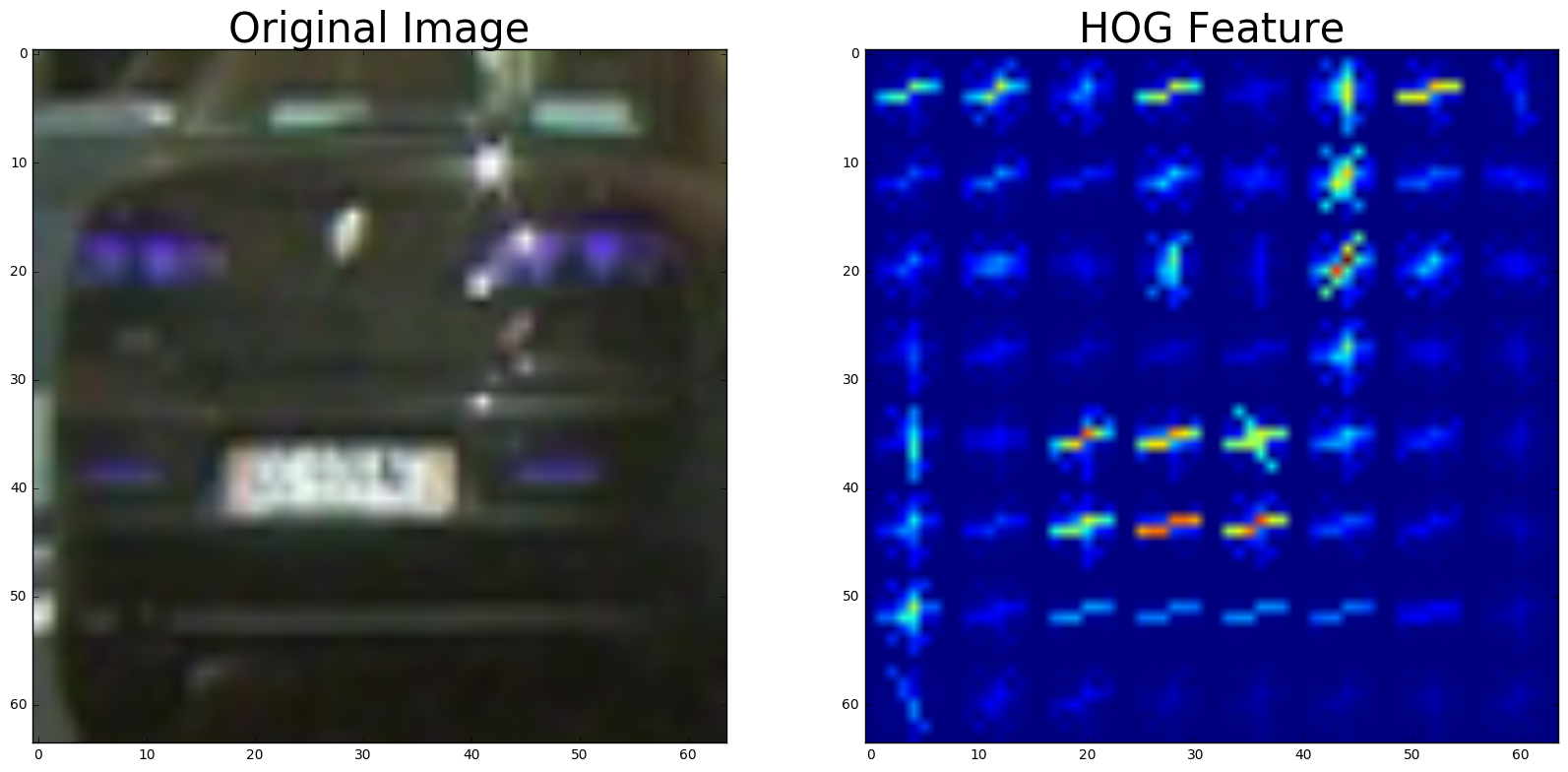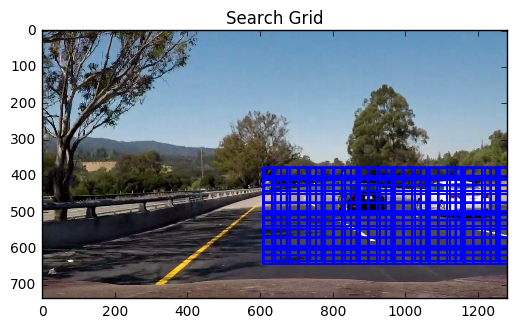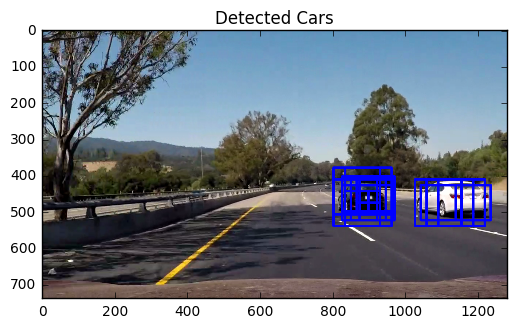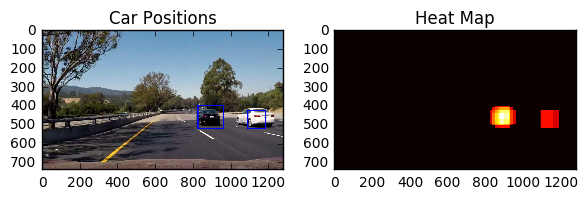Derek A site dedicated interesting things like Machine Learning and Mechatronics

# Vehicle DetectionOne of the vital portion of a self-driving car is to be able to detect other cars around it. Typically, computer vision is used to detect a car and sensor fusion with lidar/radar is used to locate the cars within the vicinity. This write-up will mainly focus on how to detect a car using computer vision. Also, one of the challenging task is to be able to detect cars quickly with minimal hardware since all the inference are done on-board.

# Solution

There are multiple ways that this problem can be solved. The three methods that I will focus on is the following:

1. SVM binary classification (detecting if a certain box is a car or is “no-car”)
2. YOLO, a single look multi-object classification algorithm
3. Semantic Segmentation, a single look algorithm that detects the “semantics” of the objects in view

You can find my final notebook results here for SVM, YOLO, and Semantic Segmentation

You can find the final video results here for SVM, YOLO, and Semantic Segmentation

## Supported Vector Machine (SVM)

Supported Vector Machine is a form of supervised machine learning (though there are unsupervised variant of SVM). The main benefits of SVM are the following:

1. Fast, you can train a decently large model with an average computer. I trained ~8K images per label (so ~16K images in total) in less than 7 seconds on an i7 (4th gen) processor. The final results had a test accuracy of 98.4%. The developers of Scikit worked really hard to do multiprocessor training hence why it’s so fast.
2. Small dataset (relatively), an accurate model can be trained with around 1000 labeled data per each label.
3. Easy to code and understand.

However, there are drawbacks to SVM which are the following

1. Multi-object classification can be tricky and hard to tune the model to get good results
2. Great for binary classification but not for detailed classification. For instance, SVM is great for determining whether an image is a dog or not a dog, but cannot predict what breed of a dog it is.
3. Does not scale with large datasets. More data after ~100000 dataset does not increase the accuracy compared to other methods like deep learning.

### Theory

The theory behind SVM is straight forward. Optimize a hyperplane that maximizes the distance between the data point and the hyperplane. A hyperplane is an mathematical object that has multiple dimensions. The final optimized hyperplane is also called the separating hyperplane. Many people like to explain it with the following:

1. 1 dimensional (D) hyperplane is a point
2. 2D hyperplane is a curve/line
3. 3D hyperplane is a surface/plane
4. Anything with more dimensions is a hyperplane

SVM does not optimize the hyperplane by calculating the distance from the data point to hyperplane. One of the clever aspect of SVM is that it optimizes the margin of the hyperplane as shown below. A hyperplane is chosen and an offset is created from it. The region between the offset is a “no datas-land” where no data can be within this area. The larger the margin of the hyperplane without any data within the margin, the better the hyperplane.Credit: Wikipedia

You might notice at this point, there is a huge limitation with SVM with the way it is currently explained. If everything I said is true, SVM will only work on linear data! This is not very useful for many modern applications. As with most algorithm that only work with linear data, the way to transform SVM to work with non-linear data is to use the kernel trick.

The kernel trick is important is because it takes the non-linear data and runs it through a function (a kernel) that transforms the data to linear through some sort of mapping or projection. A visual explanation is shown below. You can see how a parabolic surface can be projected to a 2D plane. Once the project is done, the hyperplane is pretty obvious where we can separate the purple and red data points.Credit: Wikipedia

Note, the selection of the kernel is vital to a good model. Most of the predefined kernels are determined through a series of mathematical proofs by solving eigenproblems and convex optimization (surface optimization). When working with non-linear data, different kernels should be chosen and compared to see which one makes sense. One of the most popular and robust kernel for non-linear data is the Radial Basis Function Kernel. More about the effects of non-linear kernels and choices can be found through sci-kit’s documentation which you can read here and here.

## SVM Classification on Cars in Python

To classify labels with SVM, you cannot simply just load the image like CNN (convolution neural networks). A RGB array is not detailed enough for SVM and will give you horrible results. Before the recent development of neural networks and deep learning, diligent image processing would be required before you feed it to a classification model. For image application, you have to do the following step:

1. Scale and crop each image to have the same size, color space and normalize the image.
2. Run some sort of feature descriptor. In our case, we will be using histogram of oriented gradients (HOG)
3. Feed the processed images to a SVM model
4. Bundle the results into one box

For my code, I use Scikit for the SVM model and HOG. I use numpy and OpenCV for image import and processing. More details can be found in my notebook.

### Image size, color space, normalize

The images that I run in my model are already sized to be the same. Before I run the images to the HOG, I first iterate through all the images to covert all the images to the color space I specify. OpenCV typically imports the images in BGR colorspace. I converted all the images to RGB for easier export and understanding since I normally work in RGB.

``````1
2
3
4
5
6
7
8
9
10
11
if color_space != 'RGB':
if color_space == 'HSV':
feature_image = cv2.cvtColor(image, cv2.COLOR_BGR2HSV)
elif color_space == 'LUV':
feature_image = cv2.cvtColor(image, cv2.COLOR_BGR2LUV)
elif color_space == 'HLS':
feature_image = cv2.cvtColor(image, cv2.COLOR_BGR2HLS)
elif color_space == 'YUV':
feature_image = cv2.cvtColor(image, cv2.COLOR_BGR2YUV)
elif color_space == 'YCrCb':
feature_image = cv2.cvtColor(image, cv2.COLOR_BGR2YCrCb)
``````

As for normalization, Scikit aready takes care of that for you. By default, the normalization parameter is `transform_sqrt`.

### Histogram of oriented gradients (HOG)

The name of HOG gives away what it does. PyImageSearch does a great explanation here. In order to extract the features you need, the following steps need to be followed:

1. Split the image into three color channels
2. Compute the gradient for each channel
3. Define a spatial size (essentially a sliding box size) and run through each channel, computing the histogram of the gradient magnitude of each sliding box

Splitting the image into three color channels can be done through numpy by `img[:, :, 0]` where 0 can be either 0, 1, and 2, which corresponds to red, green, and blue channels.

The remainder two steps are done automatically through the scikit toolbox. To elaborate, the gradient is simply the change in intensity of the color channel. Note, this is important because large gradient( or large change of pixel intensity) usually signify important features such as edges. After the gradient is calculated, a sliding box runs through the gradient matrix and determines the histogram (distribution of frequency of intensity values). Sometimes this sliding box will have some sort of moving weighted average to make the results more stable. After the histogram for each channel is calculated, each histogram channel is flattened and all three flattened matrix is combined using numpy’s concatenate. The final feature vector ends up being 2580 in length. This feature vector can be larger or smaller depending on how you change the spatial box size. However, once you have enough features where you get good performance, more detailed grid does not give you better results.

The final result would be something like shown below. Note, HOG results are really hard to present with simply images. Therefore, the image below is the best presentation for some basic understanding. A portion of my code is shown below as well. Once again, reference the notebook for more detail.``````1
2
3
4
5
6
7
8
9
10
11
12
13
14
15
16
17
18
19
20
21
22
23
24
25
26
27
28
29
30
31
32
33
34
def get_hog_features(img, orient, pix_per_cell, cell_per_block,
vis=False, feature_vec=True):
# Call with two outputs if vis==True
if vis == True:
features, hog_image = hog(img, orientations=orient,
pixels_per_cell=(pix_per_cell, pix_per_cell),
cells_per_block=(cell_per_block, cell_per_block),
transform_sqrt=False,
visualise=vis, feature_vector=feature_vec)
return features, hog_image
# Otherwise call with one output
else:
features = hog(img, orientations=orient,
pixels_per_cell=(pix_per_cell, pix_per_cell),
cells_per_block=(cell_per_block, cell_per_block),
transform_sqrt=False,
visualise=vis, feature_vector=feature_vec)
return features

def bin_spatial(img, size=(32, 32)):
color1 = cv2.resize(img[:, :, 0], size).ravel()
color2 = cv2.resize(img[:, :, 1], size).ravel()
color3 = cv2.resize(img[:, :, 2], size).ravel()
return np.hstack((color1, color2, color3))

def color_hist(img, nbins=32):  # bins_range=(0, 256)
# Compute the histogram of the color channels separately
channel1_hist = np.histogram(img[:, :, 0], bins=nbins)
channel2_hist = np.histogram(img[:, :, 1], bins=nbins)
channel3_hist = np.histogram(img[:, :, 2], bins=nbins)
# Concatenate the histograms into a single feature vector
hist_features = np.concatenate((channel1_hist, channel2_hist, channel3_hist))
# Return the individual histograms, bin_centers and feature vector
return hist_features
``````

### SVM with Scikit

Once you have all the required features, running the SVM classifier with Scikit is straight forward. Load the model and fit it. Since Scikit has some predefined pipeline already, not much besides this will need to be done.

``````1
2
3
4
5
6
7
8
9
10
11
12
# Use a linear SVC
svc = LinearSVC()
# Check the training time for the SVC
t = time.time()
svc.fit(X_train, y_train)
pickle.dump(svc, open("svc.p", "wb"))
t2 = time.time()
print(round(t2 - t, 2), 'Seconds to train SVC...')
# Check the score of the SVC
print('Test Accuracy of SVC = ', round(svc.score(X_test, y_test), 4))
# Check the prediction time for a single sample
t = time.time()
``````

### Sliding Window to Detect Cars

To detect a car within a certain image, you will need to create a sliding box throughout the whole image with overlapping area, cutting the image into multiple small images. To reduce the computational load, a couple of techniques can be implemented.

1. Have smaller boxes near the horizon and larger boxes near you. Cars are usually about the same size but the further it gets from you, the smaller the scale relative to you. Instead of having the whole image being a fine grid, allocate certain area to be fine and other area to be coarse.
2. Only search certain area of the image. Especially for car detection, most of the image is either the sky or the opposite lane. Crop the search area to only the area of interests. The final grid search for my application looks like the following:You will notice that there will be a lot of overlapping solutions since the sliding window has overlapping area. The result will be similar to the following:To make the results more digestible, one method is to create a heat map. A heat map is created by marking how often each pixel ends up in a box. For instance, if a pixel overlaps with four different boxes, the pixel will be marked four. The final result will then be normalized by scaling the heat map between 0 and 1. A predetermined threshold will cut out the rest of the data and set it to zero. For instance with a threshold of 0.3, if a certain pixel has a heat map intensity of 0.25, the intensity will be set to 0. After you get the heat map, we can determine the hot area in the image and draw a bounding box around it. The final result will look similar to the following: